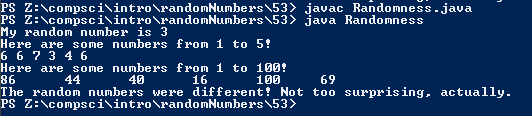# Assignemnt #53, Randomness

## Code

```      /// Name: Alex Pinder
/// Period: 5
/// Program Name: Randomness
/// File Name: Randomness.java
/// Date Complete: 12/11/15

import java.util.Random;

public class Randomness
{
public static void main ( String[] args )
{
// When a seed is placed into the random generator it displays the same numbers
Random r = new Random();

int x = 1 + r.nextInt(10);

System.out.println( "My random number is " + x );
// Without the 1 in front of each random number the range becomes 0-4 but with 3 in front it becomes 3-7
System.out.println( "Here are some numbers from 1 to 5!" );
System.out.print( 3 + r.nextInt(5) + " " );
System.out.print( 3 + r.nextInt(5) + " " );
System.out.print( 3 + r.nextInt(5) + " " );
System.out.print( 3 + r.nextInt(5) + " " );
System.out.print( 3 + r.nextInt(5) + " " );
System.out.print( 3 + r.nextInt(5) + " " );
System.out.println();

System.out.println( "Here are some numbers from 1 to 100!" );
System.out.print( 1 + r.nextInt(100) + "\t" );
System.out.print( 1 + r.nextInt(100) + "\t" );
System.out.print( 1 + r.nextInt(100) + "\t" );
System.out.print( 1 + r.nextInt(100) + "\t" );
System.out.print( 1 + r.nextInt(100) + "\t" );
System.out.print( 1 + r.nextInt(100) + "\t" );
System.out.println();

int num1 = 1 + r.nextInt(10);
int num2 = 1 + r.nextInt(10);

if ( num1 == num2 )
{
System.out.println( "The random numbers were the same! Weird." );
}
if ( num1 != num2 )
{
System.out.println( "The random numbers were different! Not too surprising, actually." );
}
}
}

```

### Picture of the output